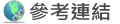## 使用 串接查詢條件

```SELECT UserID
FROM tblUser
WHERE 1=1
...
```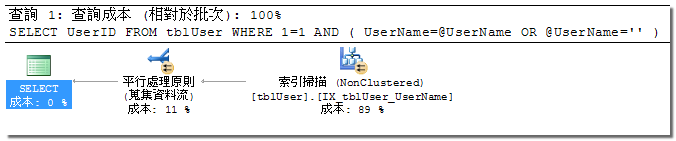```SELECT UserID
FROM tblUser
WHERE 1=1
```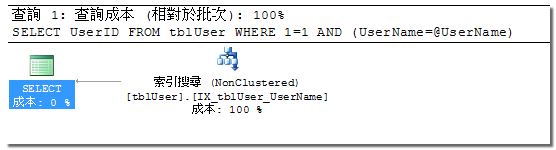```DECLARE @LocalUserName nvarchar(20);

```

## 使用 Joins

• To optimize queries, one of the first basic strategies is to minimize the number of join clauses used.
• Another consideration is that outer joins incur more cost than inner joins because of the extra work needed to find the unmatched rows.

## 使用 Sub Query

```SELECT p.ProductID, p.ProductName, p.UnitPrice
FROM Products AS p
WHERE p.UnitPrice > ( SELECT AVG(P2.UnitPrice) FROM Products P2)
```
```SELECT p.ProductID, p.ProductName, p.UnitPrice
FROM Products AS p
WHERE Exists ( SELECT ProductID FROM OrderDetails OD WHERE OD.ProductID = P.ProductID )
```

### 子查詢調校範例

```SELECT
OrderID,OrderDate,
(Select Top(1) UnitPrice FROM OrderDetails OD WHERE OD.OrderID=O.OrderID ORDER BY Quantity) as UnitPrice,
(Select Top(1) Quantity FROM OrderDetails OD WHERE OD.OrderID=O.OrderIDORDER BY Quantity) as Quantity
FROM Orders O
WHERE OrderID=10256
```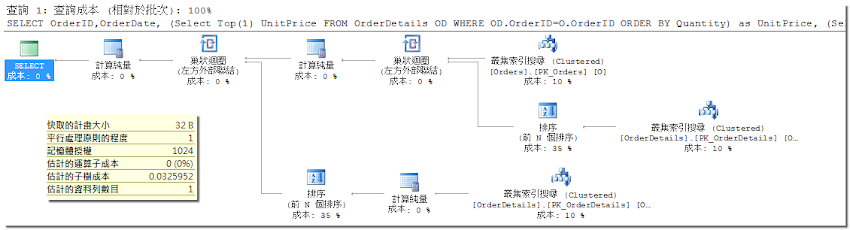```SELECT
OrderID,OrderDate,A.*
FROM Orders O
OUTER APPLY (
Select Top(1) UnitPrice,Quantity FROM OrderDetails OD WHERE OD.OrderID=O.OrderID ORDER BY Quantity
) as A
WHERE OrderID=10256
```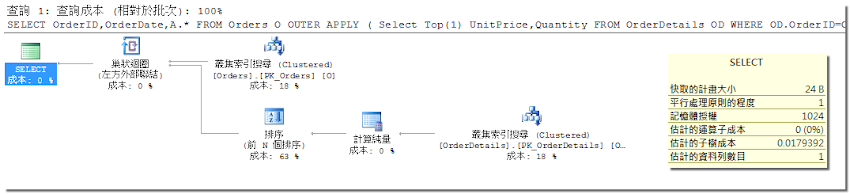```WITH A as (
SELECT O.OrderID, O.OrderDate, OD.UnitPrice, OD.Quantity,
ROW_NUMBER() OVER (PARTITION BY O.OrderID ORDER BY Quantity DESC) as RowNum
FROM OrderDetails OD
INNER JOIN Orders O ON O.OrderID=OD.OrderID
WHERE O.OrderID=10256
)
SELECT * FROM A WHERE ROwNum=1
```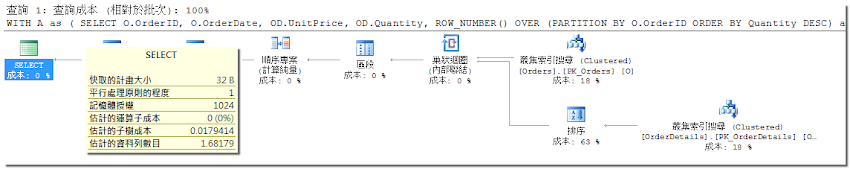## 使用 Scalar UDFs

Scalar UDFs是一種很常用的自訂函式類型，但不幸地，它也會降底執行效率。原因是這種函式無法被最佳化器擴展和最佳化到主要的查詢計畫中。執行計畫只會叫用這個函式，不會最佳化，也不會將它列入執行成本統計之中。

```SELECT OrderID, OrderDate, dbo.fuGetEmpName(1)
FROM Orders
```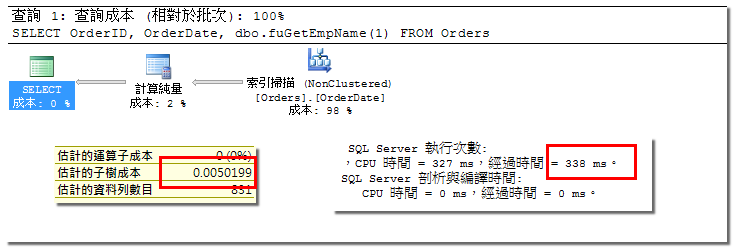```SELECT OrderID, OrderDate, ISNULL(
(
SELECT FirstName + ' ' + LastName
FROM Employees
WHERE EmployeeID = O.OrderID
FROM Orders O
```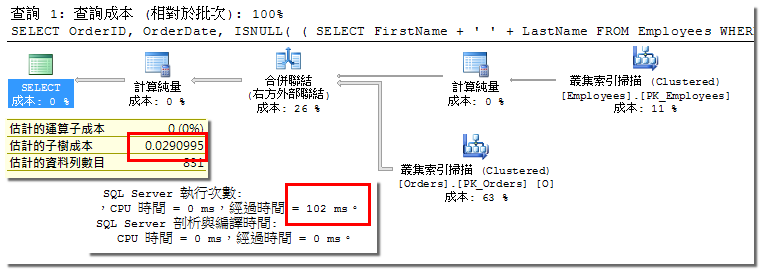## 使用 Table-Valued UDFs

Table-Valued 的 UDFs 共有三種型態，其中二種是使用 TSQL 撰寫，另一種是 CLR 語言。

• T-SQL inline table-valued UDF
• T-SQL multistatement table-valued UDF
• CLR table-valued UDF

### inline t-v UDF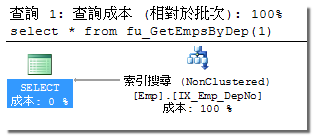### mulitstatement t-v UDF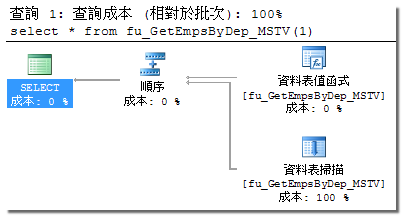## 使用 Cursors

You should try to rewrite cursor logic into one or more set-based statements (SELECT, INSERT, UPDATE, DELETE, or MERGE). If you cannot convert the cursor logic to a set-based statement, consider implementing the logic using a CLR stored procedure ora table-valued UDF instead (depending on the functionality you need).